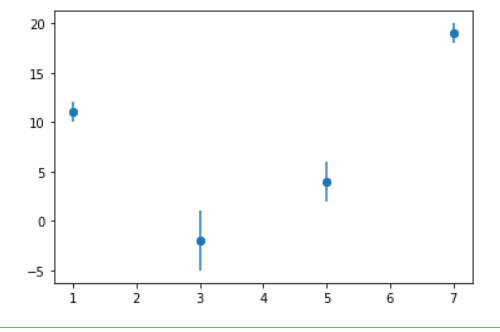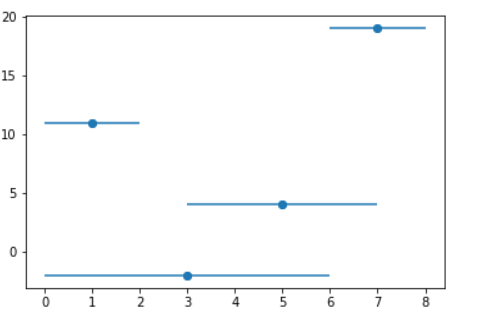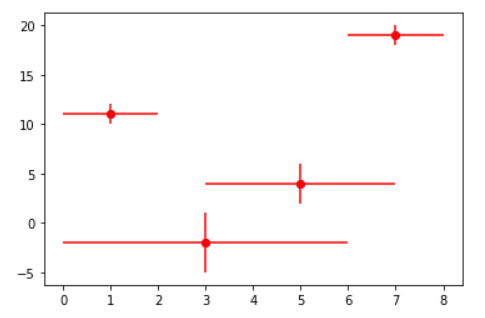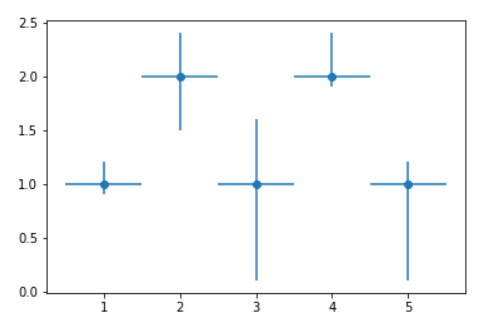Related Articles
Use error bars in a Matplotlib scatter plot
• Difficulty Level : Basic
• Last Updated : 17 Dec, 2020

Prerequisites: Matplotlib

In this article, we will create a scatter plot with error bars using Matplotlib. Error bar charts are a great way to represent the variability in your data. It can be applied to graphs to provide an additional layer of detailed information on the presented data.

### Approach

• Import required python library.
• Create data.
• Pass required values to errorbar() function
• Plot graph.

Syntax: matplotlib.pyplot.errorbar(x, y, yerr=None, xerr=None, fmt=”, ecolor=None, elinewidth=None, capsize=None, barsabove=False, lolims=False, uplims=False, xlolims=False, xuplims=False, errorevery=1, capthick=None, \*, data=None, \*\*kwargs)

Parameters: This method accept the following parameters that are described below:

• x, y: These parameters are the horizontal and vertical coordinates of the data points.
• fmt: This parameter is an optional parameter and it contains the string value.
• capsize: This parameter is also an optional parameter. And it is the length of the error bar caps in points with default value NONE.

Implementation of the concept discussed above is given below:

Example 1: Adding Some error in a ‘y’ value.

## Python3

 `import` `matplotlib.pyplot as plt`` ` `a ``=` `[``1``, ``3``, ``5``, ``7``]``b ``=` `[``11``, ``-``2``, ``4``, ``19``]``plt.scatter(a, b)`` ` `c ``=` `[``1``, ``3``, ``2``, ``1``]`` ` `plt.errorbar(a, b, yerr``=``c, fmt``=``"o"``)``plt.show()`

Output:Example 2: Adding Some errors in the ‘x’ value.

## Python3

 `import` `matplotlib.pyplot as plt`` ` `a ``=` `[``1``, ``3``, ``5``, ``7``]``b ``=` `[``11``, ``-``2``, ``4``, ``19``]``plt.scatter(a, b)`` ` `c ``=` `[``1``, ``3``, ``2``, ``1``]`` ` `plt.errorbar(a, b, xerr``=``c, fmt``=``"o"``)``plt.show()`

Output:Example 3: Adding error in x & y

## Python3

 `import` `matplotlib.pyplot as plt`` ` `a ``=` `[``1``, ``3``, ``5``, ``7``]``b ``=` `[``11``, ``-``2``, ``4``, ``19``]``plt.scatter(a, b)`` ` ` ` `c ``=` `[``1``, ``3``, ``2``, ``1``]``d ``=` `[``1``, ``3``, ``2``, ``1``]`` ` `# you can use color ="r" for red or skip to default as blue``plt.errorbar(a, b, xerr``=``c, yerr``=``d, fmt``=``"o"``, color``=``"r"``)`` ` `plt.show()`

Output:Example 4: Adding variable error in x and y.

## Python3

 `# importing matplotlib``import` `matplotlib.pyplot as plt`` ` ` ` `# making a simple plot``x ``=` `[``1``, ``2``, ``3``, ``4``, ``5``]``y ``=` `[``1``, ``2``, ``1``, ``2``, ``1``]`` ` `# creating error``y_errormin ``=` `[``0.1``, ``0.5``, ``0.9``,``              ``0.1``, ``0.9``]``y_errormax ``=` `[``0.2``, ``0.4``, ``0.6``,``              ``0.4``, ``0.2``]`` ` `x_error ``=` `0.5``y_error ``=` `[y_errormin, y_errormax]`` ` `# ploting graph``# plt.plot(x, y)``plt.errorbar(x, y,``             ``yerr``=``y_error,``             ``xerr``=``x_error,``             ``fmt``=``'o'``)  ``plt.show()`

Output:Attention geek! Strengthen your foundations with the Python Programming Foundation Course and learn the basics.

To begin with, your interview preparations Enhance your Data Structures concepts with the Python DS Course. And to begin with your Machine Learning Journey, join the Machine Learning – Basic Level Course

My Personal Notes arrow_drop_up